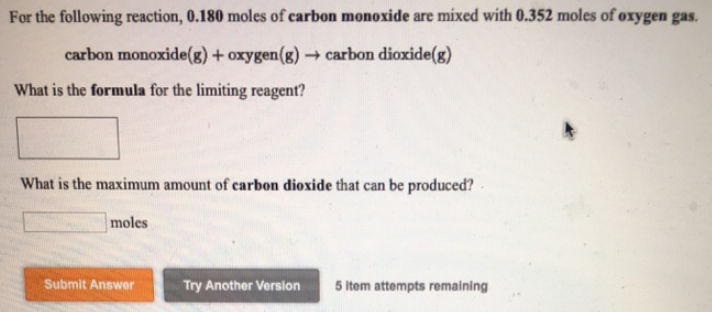# Problem: For the following reaction, 0.180 moles of carbon monoxide are mixed with 0.352 moles of oxygen gas. carbon monoxide (g) + oxygen (g) → carbon dioxide (g) What is the formula for the limiting reagent? What is the maximum amount of carbon dioxide that can be produced?

###### FREE Expert Solution
92% (2 ratings)###### Problem Details

For the following reaction, 0.180 moles of carbon monoxide are mixed with 0.352 moles of oxygen gas.

carbon monoxide (g) + oxygen (g) → carbon dioxide (g)

What is the formula for the limiting reagent?

What is the maximum amount of carbon dioxide that can be produced?